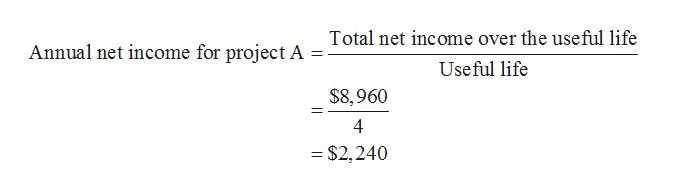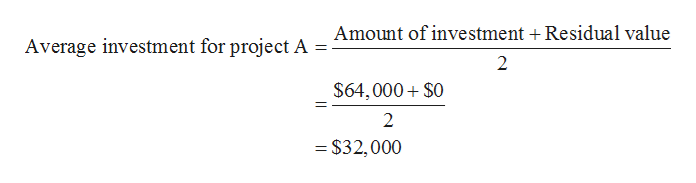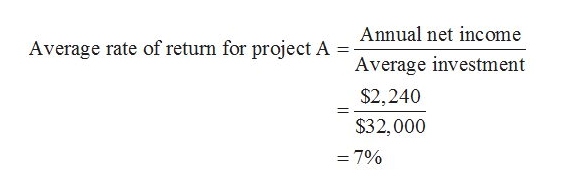# The following data are accumulated by Watershed Inc. in evaluating two competing capital investment proposals: Project AProject ZAmount of investment\$64,000  \$84,000 Useful life4 years  9 years Estimated residual value0  0 Estimated total income over the useful life\$8,960  \$43,470 Determine the expected average rate of return for each project. Round your answers to one decimal place.Project A%Project Z%

Question
82 views

The following data are accumulated by Watershed Inc. in evaluating two competing capital investment proposals:

 Project A Project Z Amount of investment \$64,000 \$84,000 Useful life 4 years 9 years Estimated residual value 0 0 Estimated total income over the useful life \$8,960 \$43,470

Determine the expected average rate of return for each project. Round your answers to one decimal place.

 Project A % Project Z %
check_circle

Step 1

Hence, the annual net income for project A is \$2,240. It is obtained by dividing total net income over the useful life by useful life.help_outlineImage TranscriptioncloseTotal net income over the useful life Annual net income for project A Useful life \$8,960 4 = \$2,240 fullscreen
Step 2

Hence, the average investment for project A is \$32,000. It is obtained by dividing the sum of amount of investment and residual value by 2.help_outlineImage TranscriptioncloseAmount of investment + Residual value Average investment for project A = %3D \$64,000+ \$0 = \$32,000 fullscreen
Step 3

Hence, the average rate of return for project A is 7%. It is obtain...help_outlineImage TranscriptioncloseAnnual net income Average rate of return for project A Average investment \$2,240 \$32,000 = 7% %3D fullscreen

### Want to see the full answer?

See Solution

#### Want to see this answer and more?

Solutions are written by subject experts who are available 24/7. Questions are typically answered within 1 hour.*

See Solution
*Response times may vary by subject and question.
Tagged in